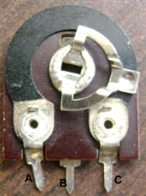## How to use a preset?

There are 3 pins/terminals on a preset. The maximum resistance that a preset can provide is written on it. If 100K is written on preset, it means that we can vary its resistance from 0 Ohm to 100K. A movable metal is rotated in clockwise or anticlockwise direction that changes the resistance of preset.

Now, we name the three terminals as A,B, C.

• If we take terminal A and terminal B, and rotate the movable metal in clockwise direction, the resistance of preset increases from 0 to maximum. As we move the metal in anticlockwise direction, the resistance decreases.
• If we take terminal A and terminal C, and rotate the movable metal in anticlockwise direction, the resistance of preset increases from 0 to maximum. As we move the metal in clockwise direction, the resistance decreases.

## How to use a potentiometer?

• 1. If we take terminal  B and terminal C, and rotate the knob in clockwise direction, the resistance of potentiometer increases from 0 to maximum. As we move the knob in anticlockwise direction, the resistance decreases.
• 2. If we take terminal A and terminal B, and rotate the knob in anticlockwisedirection, the resistance of potentiometer increases from 0 to maximum. As we move the knob in clockwise direction, the resistance decreases.

## How to use a trimmer potentiometer?• 1. If we take lead  A and lead B, and rotate the knob in clockwise direction, the resistance of potentiometer increases from 0 to maximum. As we move the knob in anticlockwise direction, the resistance decreases.
• 2. If we take terminal B and terminal C, and rotate the knob in anticlockwise direction, the resistance of potentiometer increases from 0 to maximum. As we move the knob in clockwise direction, the resistance decreases.